# Valuation of Securities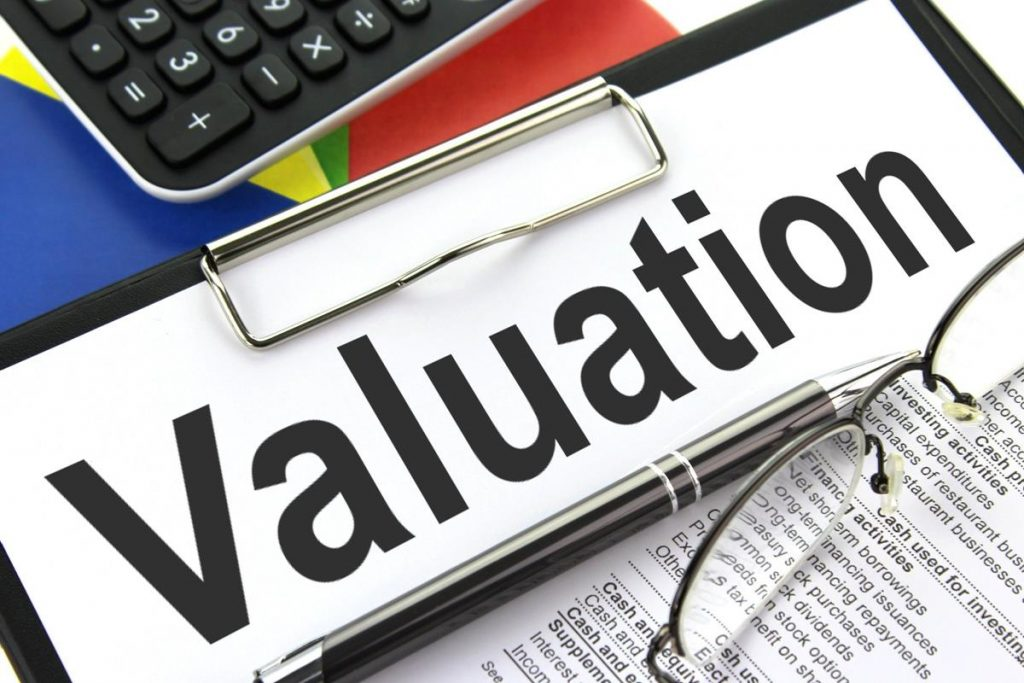There are various pricing models used for security valuation. These can be based on the balance sheet of the organisation or discounted cashflows. In other cases, ratios can be applied. Valuation is done in order to determine if a security is undervalued, overvalued or to monitor the overall performance of a security. In some cases, security valuation is done to determine whether a profit can be made in the event of a takeover. Some of the popular methods of security valuation are described as follows.

## Balance sheet valuation approach

Under the balance sheet, there are 3 methods of valuation of securities; the book value, liquidation value and the replacement cost method.

### Book Value Per Share

The book value per share is also called the net asset value per share. It simply shows the net worth of a share based on a company’s balance sheet.

Formula

The book value per share = (Assets – Liabilities)/Number of shares in Issue or = (Shareholders funds)/Number of shares in Issue

For example; suppose the table below has figures extracted from the balance sheet of a company

 Assets \$ 7 million Liabilities \$ 2 million Shareholders’ Funds \$ 5 million Issued share Capital 4 million

The book value per share = (7-2)/4 = \$1.25

Or   5/4 = \$1.25

• This valuation means that the ordinary share’s book value is \$1.25, any price above it indicates that it is overvalued, a price below indicates that the share is undervalued.
• This method of valuation may not reflect the true value of a share since the assets and liabilities of a firm are not valued at market prices but based at historical costs.

### Liquidation value

The liquidation value is used to identify companies for takeover or liquidation. That way, profit can be obtained from the difference between the market price and the liquidation price. Since the valuation of assets and liabilities is not easy a strict valuation in undertaken and a portion of the value is cut off to allow for errors.

For example, a company can be taken over at a price of \$1 per share.

 Value of assets 6 million Value of liabilities 1,2 million Number of shares 4 million Price paid per share \$1

The profit obtained will be;

[(Value of Assets – Value of Liabilities)/Number of shares in Issue] – Price paid per share

[(6-1.2)/4]-1 = \$0.20 per share

Therefore, the total profit for 4 million shares will be (4 000 000*0.20) \$800 000

### The replacement cost

The replacement cost method of valuation of securities is based on the replacement cost of a company’s assets and liabilities. This method is based on the fact that the market value of a company cannot be higher than the cost of its assets and liabilities otherwise competitors will enter that line of business. When that happens, the market value of the business is pushed downwards, lowering the profits and increasing the supply of shares in the market. At the same time the replacement value of the company’s assets and liabilities will go up until the market value of the company is close to the replacement cost.

Tobin’s  , which is the ratio of market value to replacement cost, will tend toward 1 in the long run and may be different from 1 in the short run.

## Discounted cash flow approach

Discounted cash flow methods discount dividends and coupon payments in order to determine the value of securities.

### Present value valuation approach

For Bonds, the present value is used to determine the value of the security. Thus, the value of a bond will be obtained from discounting the coupon payments over the term of the bond and adding the principal amount repayable at maturity.

Therefore, the price of the bond is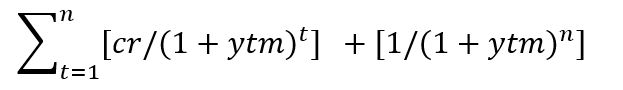Where cr = coupon rate

ytm = yield to maturity

n = number of periods or years

In the case of a perpetual bond or perpetual preference share the valuation or price is determined by;

Price of  a perpetual bond  = (fixed coupon rate)⁄ (ytm (required rate))

Price of a perpetual preference = (fixed dividend rate)⁄(required rate)

The above formulas are obtained from the present value formula below;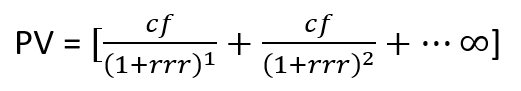Where cf = annual cashflow (interest payments or dividends)

rrr = required rate of return

### Dividend Discount model (DDM)

Ordinary shares are like perpetual bonds only that they do not have a fixed dividend. Also, with equities there is an infinite period of investment therefore, there is no face value to be repaid. Thus, the valuation of ordinary shares is obtained the same way perpetual preference shares are obtained. Only that D = dividends are written in place of cf (cash flow). The valuation of ordinary shares is the present value of dividends and this method is referred to as the dividend discount model.

The price of ordinary shares = PV = D/rrr

Also, obtained from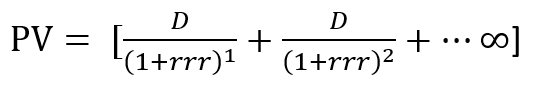### Gordon constant growth dividend discount model (GCGDDM)

This method of security valuation takes the constant growth dividend discount model and applies a constant growth rate of dividends in the future. In addition, the Gordon constant growth dividend discount model is useful in forecasting future dividends.

Formulae for the dividend discount model (DDM) in the case of growing dividends;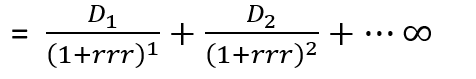The formula above is made practical by assuming that the dividends will grow at a constant rate in the future. Therefore, Gordon constant growth dividend discount model is calculated as follows;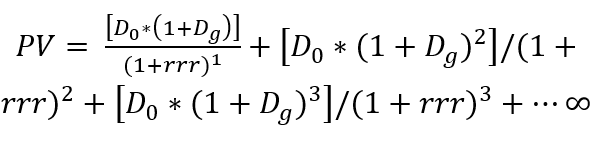Where;

Do = past dividend

Dg = assumed growth rates in dividends

rrr = required rate of return

The formula simplifies to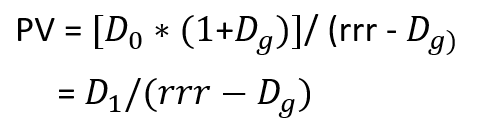The present value of the share will be higher as the required rate of return falls and if the growth rate in dividends is higher.

### Multistage Growth Model

Smaller companies have dividends that grow rapidly in the early years unlike companies that have been operating for a while. The valuation of securities can be done using a multistage growth model. The various growth rates in dividends can be written in a table and the PV formula can be applied.

## Other valuation methods

Security valuation can be done using various methods. Some of the other methods are summarised below;

• Price earnings ratio

This is one of the oldest ways of security valuation. It is also called the price earnings multiple. (m)

m = Po/EPS

where Po = price of the share at time zero

EPS = earnings per share

• Price cash flow ratio

Some analyst prefer to make use of the price cash flow ratio because EPS can be manipulated. It is more difficult to manipulate cash flows

P/CF = P/Cf

Where P = Price of the share

Cf = Cash flow per share expected in the next accounting period

• Price book value ratio

This method of security valuation is simply the price per share to the book value per share

P/BV = P/BVf

where;

P = Price of the share

BVf =  expected book value at the end of the financial year

These methods of security valuation are not exhaustive, there are many other methods such as price sales ratio as well as the free cash flow approach that can be applied. Each method has its advantages and drawbacks so, it is up to the analyst to decide the best possible methods for the valuation of securities.